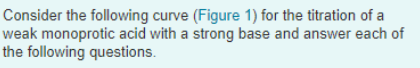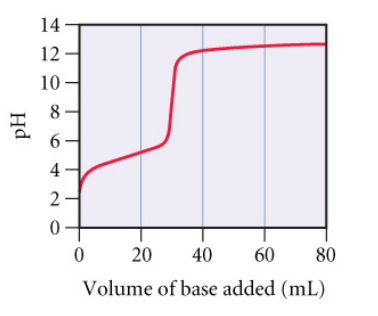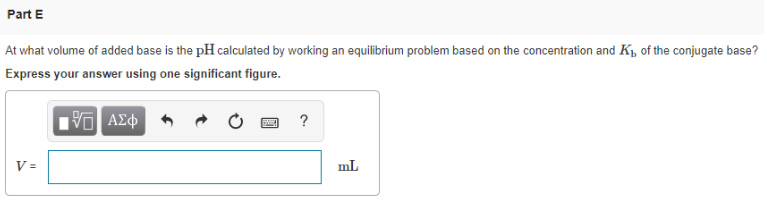# Consider the following curve (Figure 1) for the titration of a weak monoprotic acid with a strong base and answer each of the following questions. At what volume of added base is the pH calculated by working an equilibrium problem based on the concentration and Kb of the conjugate base? Express your answer using one significant figure.